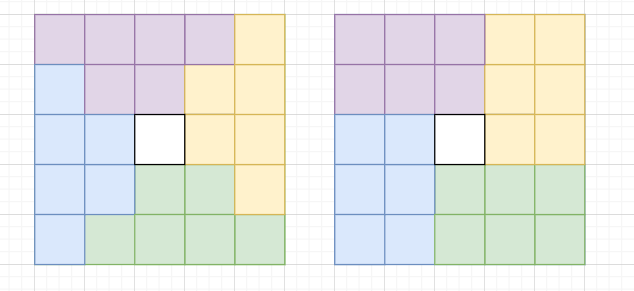# 原地算法的经典题目

### 部分场景

#### 1.数组翻转

``````template<typename T>
function reverse-in-place(a[0..n])
for i from 0 to floor(n/2)
tmp := a[n-i]
a[i] := a[n-i]
a[n-i] := tmp
``````

#### 2.矩阵旋转

https://leetcode-cn.com/problems/rotate-matrix-lcci/``````void rotate(vector<vector<int>>& matrix) {
int N=matrix.size(),temp;
for(int row=0;row<N/2;row++){
for(int col=row;col<=N-row-2;col++){
temp=matrix[row][col];
matrix[row][col]=matrix[N-col-1][row];
matrix[N-col-1][row]=matrix[N-row-1][N-col-1];
matrix[N-row-1][N-col-1]=matrix[col][N-row-1];
matrix[col][N-row-1]=temp;
}
}
}
``````

#### 3.二叉树转链表

``````/**
* Definition for a binary tree node.
* struct TreeNode {
*     int val;
*     TreeNode *left;
*     TreeNode *right;
*     TreeNode() : val(0), left(nullptr), right(nullptr) {}
*     TreeNode(int x) : val(x), left(nullptr), right(nullptr) {}
*     TreeNode(int x, TreeNode *left, TreeNode *right) : val(x), left(left), right(right) {}
* };
*/
void flatten(TreeNode* root) {
TreeNode *cur = root;
while (cur != nullptr) {
if (cur->left != nullptr) {
TreeNode* next = cur->left;
TreeNode* predecessor = next;
while (predecessor->right != nullptr) {
predecessor = predecessor->right;
}
predecessor->right = cur->right;
cur->left = nullptr;
cur->right = next;
}
cur = cur->right;
}
}
``````

#### 最大子数组和

https://leetcode-cn.com/problems/maximum-subarray/

``````int maxSubArray(vector<int>& nums) {
int pre = 0, maxAns = nums;
for (const auto &x: nums) {
pre = max(pre + x, x);
maxAns = max(maxAns, pre);
}
return maxAns;
}

``````